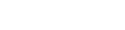• Home
• IPMAT Question Paper...

# IPMAT Question Paper Analysis & Solutions

Author : Palak Khanna

January 27, 2023

SHARE

The Indian Institute of Management (IIM) conducts the Integrated Program in Management Aptitude Test (IPMAT) annually for providing admission to the five-year integrated program in management.

Going through the previous year's paper analysis will let you understand better the essential topics to focus on more for better results.

Our experts at Supergards have curated the IPMAT Exam Analysis and Solutions of the past five years in this post.

The takeaways from this post can immensely help you in streamlining your preparation to a result-oriented pathway and also get an idea about the number of questions asked from each subject, the difficulty level of the paper, type of questions, and more.

## IPMAT Exam Analysis Trend

The following points explain the Past Five-year's Exam Analysis of the IIM IPM Exam, such as mode of exam, sections involved, nature of questions, and more.

• Type of questions: Multiple Choice Questions MCQs and Short Answer (SA) questions.
• Sections: Generally, there are three sections in the exam. Two sections are of quantitative ability, and one is verbal ability.
• Maximum Marks: The is conducted for a total of 400 marks.
• Marking scheme: 4 marks are awarded for every correct answer, one mark is deducted for every wrong answer in MCQs, and there is no negative marking in short answers.

• Sectional Cut-off: It is applicable to all three sections.
• Difficulty Level: The overall difficulty level of IPMAT, considering previous years’ examination, is moderate to difficult.
• Verbal Ability: This is the most scoring section in the examination, with about 70-80% of questions from easy to moderate difficulty.
• Quantitative Ability: MCQ is generally the most challenging section, with about 40-50% moderate to complex questions.

### IPM Question Paper Analysis for Quantitative Ability (Short Answers)

As per the Exam Pattern for IPMAT, 50% of the question paper comprises the quantitative section (including MCQs and short answer-type questions).

• Generally, the SA section is of moderate difficulty having 20-30% tricky questions.
• Majorly, the questions are based on Logs, Arithmetic Progressions, Geometry, P&C, Probability.
• Statistically, 40% of questions are based on logarithms and Sequence & Series, and the same trend is followed from the past few years.
• A good attempt would be anything between 70-80% attempt with 80% accuracy.
• The pie chart plotted below represents an overall trend of the percentage of questions asked from different topics.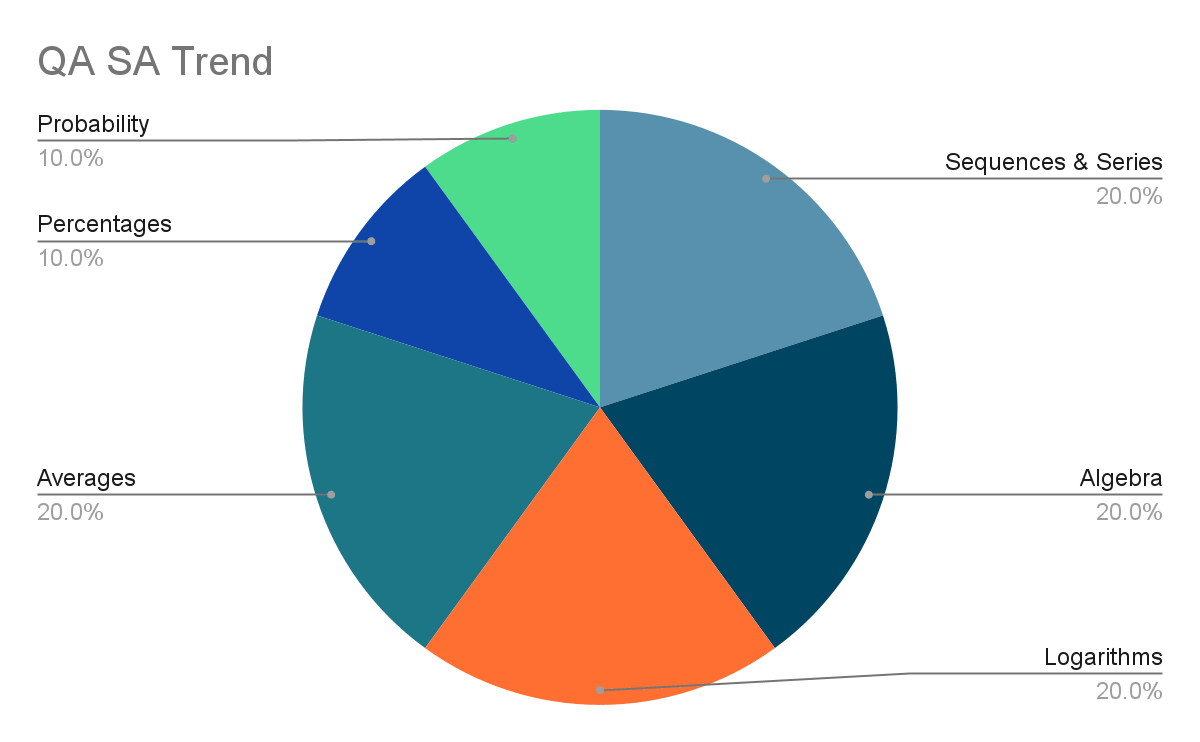Read more: Important topics for the IPMAT exam

### IPMAT Question Paper Analysis for Quantitative Ability (MCQ) Section

• Generally, this section is the most difficult section having 40-50% tricky questions and 40 questions to be completed within 40 minutes.
• Majorly questions are based on Number Systems, Arithmetic, Algebra, Geometry and Menstruation, P & C, Sets, Data Interpretation, and Matrices.
• Statistically, 50-55% of questions are based on Algebra, Arithmetics, and Data Interpretation.
• A good attempt would be anything between 65-70% attempt with 85% accuracy, fetching you about 80-90 marks.
• The below pie chart represents an overall trend of the percentage of questions asked from different topics.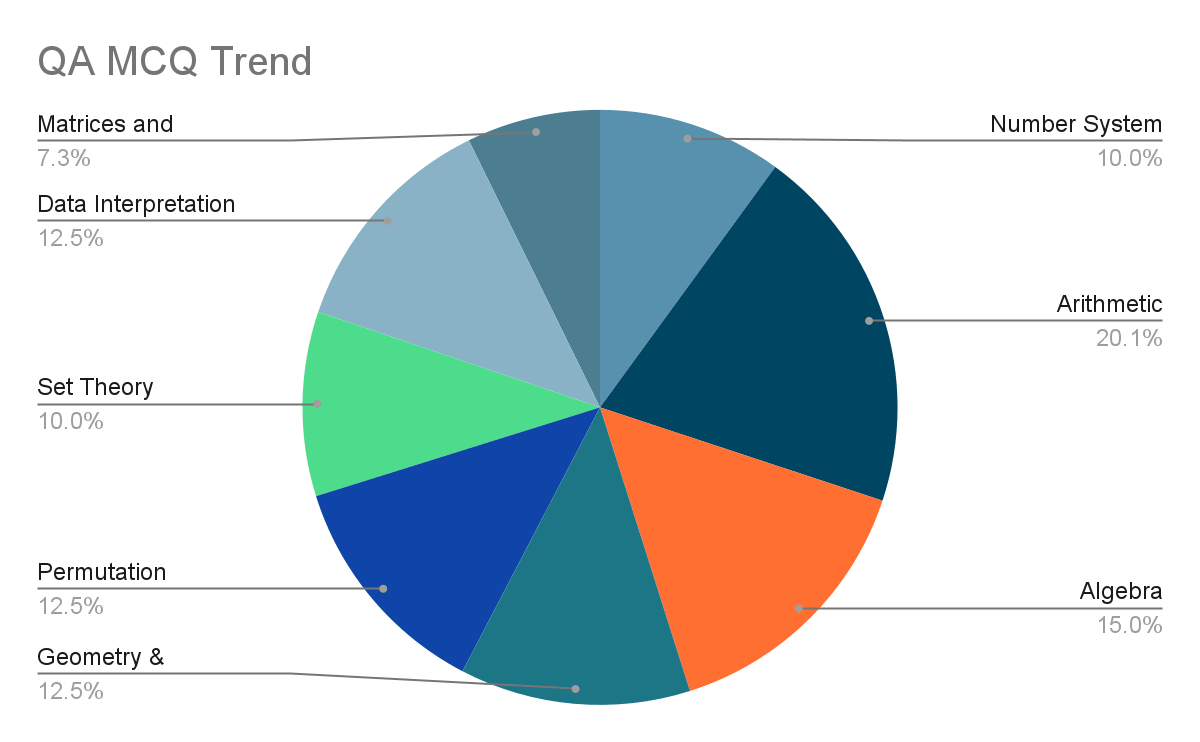### IPM Question Paper Analysis for Verbal Ability Section

As per the previous year's trends, the IPMAT verbal ability section questions do not test your conventional English language or do not ask direct vocabulary questions like antonyms, synonyms, analogies, etc.

Instead, the verbal questions test your overall expertise in English with question types like usage of words & phrases, fill in the blanks, and your capability to use contextual vocabulary.

Check out the detailed IPMAT Question Paper analysis and solutions for the verbal ability section from the post below.

• Generally, this section is the easiest section having about 80% of questions of easy to moderate level difficulty.
• Majorly questions are based on Reading Comprehension, Fill in the blanks, Sentence Completion, Para Jumbles, and sentence completion-based questions.
• Statistically, 50-55% of questions are based on reading comprehension and fill-in blanks.
• A good attempt would be anything between 90% attempt with 85-90% accuracy, fetching you about 120-125 marks.
• The below pie chart represents an overall trend of the percentage of questions asked from different topics.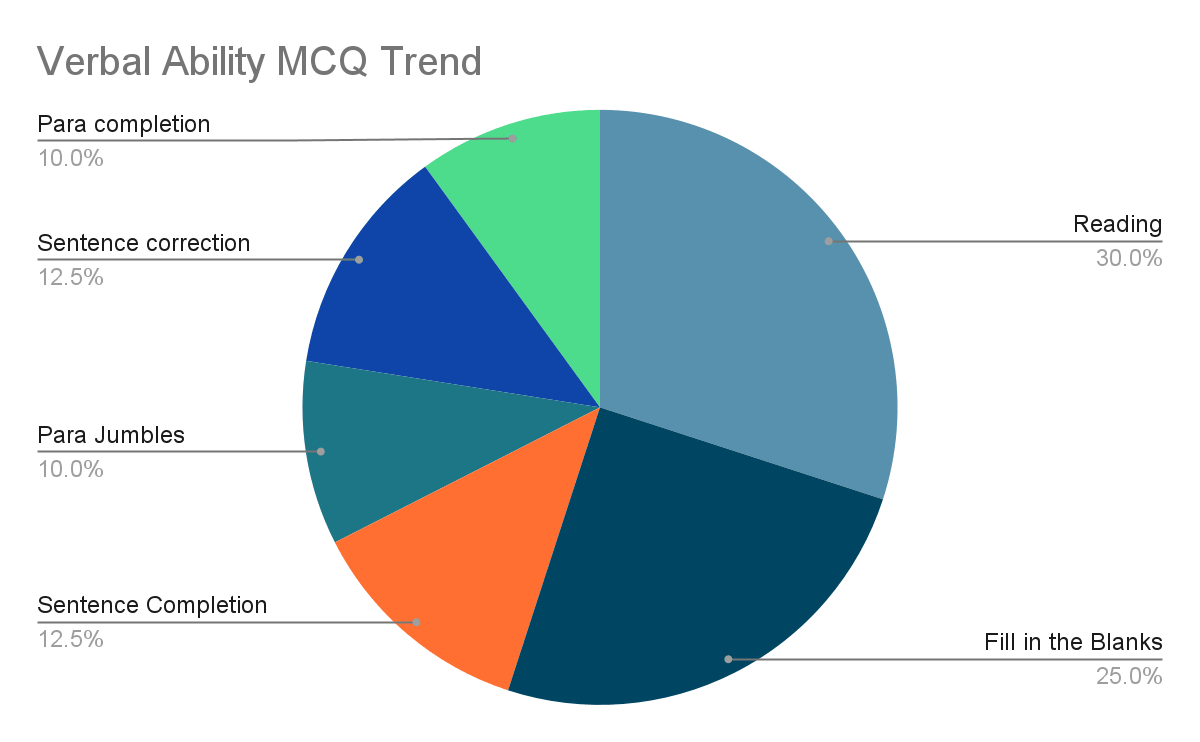Read more: IPMAT Fee structure and scholarships

### Important Questions with Solutions for IPMAT Quantitative Ability (Short Answer)

To help you get an idea about the type of questions asked in the quantitative aptitude (short answer) section, we have provided a few sample questions that were curated from the previous year's  IPMAT question papers.

Try to solve them for a better understanding of the difficulty level and the type of questions asked in the exam.

Question 1: In a division problem, the product of quotients and the remainder is 24 while their sum is 10. If the divisor is 5 then the dividend is __________. (IPMAT 2020)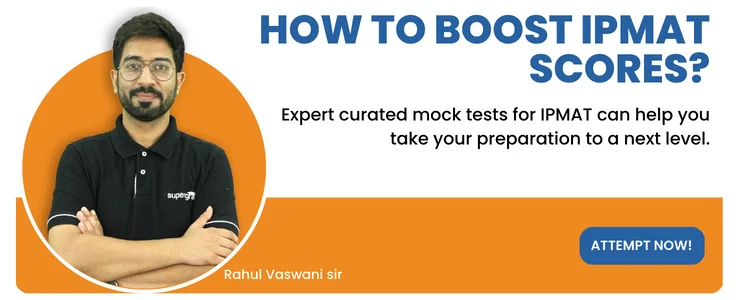Solution 1 :

In this problem, we have given that the product of quotient(q) and the remainder(r) is 24.   i.e q x r = 24 -( i )

And it is also given that their sum is 10   i.e q + r = 10 -( ii )

Divisor(d) = 5.

( i ) and ( ii ) represent the sum and product of a quadratic equation, from ( ii ) putting r = q - 10, in ( i ), we will get q x (10 - q) = 24,    i.e  q2-10q + 24 = 0, which will give

q = 6 or 4.

Therefore one of them will be 6, and the other one will be 4. We do not know yet whether the quotient is 6 and the remainder is 4 or vice versa.  We know that  Dividend(n) = divisor(d) x quotient(q) + remainder(r) .

From the given data, n = 5 x q + r. - ( iii ),

As the divisor is 5, we infer that the remainder obviously will be smaller than 5. Therefore, the remainder will be 4, and the quotient will be 6. Putting the above-decided value in ( iii ).    n = 5(6) + 4 , therefore n = 34.

Question 2 : The shortest distance from the point (-4,3) to the circle x2 + y2 = 1 is __________. ( IPMAT 2020 )

Solution 2.

In this question, we have been given the equation of a circle and the coordinates of a point.

From the standard equation of circle i.e x2 + y2 = a2, we have a = 1.

The shortest distance of the point P(-4, 3) will be the distance from point P to the point where a straight line drawn from P to the circle's origin intersects the circle. As shown in the figure below, the shortest distance is PM.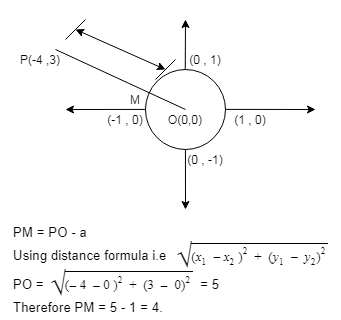Question 3: The value ofQuestion 4: The minimum value of f(x)=|3-x|+|2+x|+|5-x| is equal to __________.

Solution.

Form f(x)=|3-x|+|2+x|+|5-x| We know that critical points would be x = 3 , x = -2 , and x = 5.

The slope of the line changes at these critical points.

Example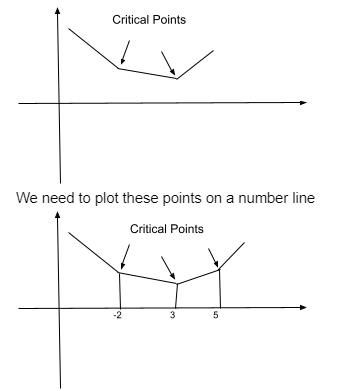Now we need to select the critical point which has the lowest value of y axis, from the figure above f(3) will have the lowest value.

f(3) = |3 - 3|+|2 + 3|+| 5 - 3|

f(3) = 0 + 5 + 2 .

f(3) = 7.

Question 5: Ashok purchased pens and pencils in the ratio 2: 3 during his first visit and paid Rs. 86 to the shopkeeper. During his second visit, he purchased pens and pencils in the ratio of 4: 1 and paid Rs. 112. The cost of a pen, as well as a pencil in rupees, is a positive integer. If Ashok purchased four pens during his second visit, then the amount he paid in rupees for the pens during the second visit is __________. (IPMAT 2020)

Solution.

We have been given that when the person spends Rs. 86, the ratio of pen and pencil is 2:3.

And when he pays Rs. 112, the ratio of pen and pencil is 4:1. And in this visit, the total number of pens is 4.

Let x be the price per pen and y be the price per pencil.

Therefore we have

4x + y = 112

2x + 3y = 86 (From first visit)

It is in Ratios, so it can be 4x + 6y = 86 (or)

6x + 9y = 86 (or)

8x + 12y = 86 and so on.

As we know, 4x + y = 112, we can infer that 4x + 6y = 86 is not possible, and hence all the equations after this are not possible.

So now we have 4x + y = 112

2x + 3y = 86

Solving the above equation we will get y = 12.

Therefore 4x + 12 = 112

=> 4x = cost of pens = 112 -12 = 100

Question 6: In a four-digit number, the product of thousands digit and units digit is zero while their difference is 7. The product of the middle digits is 18. The thousands digit is as much more than the units digit as the hundreds digit is more than the tens digit. The four-digit number is __________.

Solution.

Let the four-digit number be ABCD. D is the unit place number, C is the tens digit number, B is the hundreds digit number, and A is the thousand digit number.

Given data

1.  A x D = 0

This implies one of them will be zero; as A is the rightmost place, its value cannot be zero.

Therefore D = 0.

1. A - D = 7

As D = 0, A will be 7.

1. B x C = 18

B and C can hold values from 0-9 only; looking at the possible combinations, BC can be 92( or 29) or 63(or 36).

1. Given that the AD difference is the same as the difference of BC, therefore, B is 9 and C is 2.

Question 7: Two friends run a 3-kilometer race along a circular course of length 300 meters. If their speeds are in the ratio 3:2, the number of times the winner passes the other is __________.

Solution.

Let the speed of the fast one be 3x, and the speed of the slow one be 2x.

Time required for the fast one to cover one complete round = tf = 300/3x

Time required for the slow one to cover one complete round = ts = 300/2x

For finding out the time at which both meet for the first time, we need to find lcm of tf and ts

Tmeet = 300/x (lcm of tf and ts)

Distance traveled by the fast one when they meet = Tmeet * speed of fast one

= 300/x * 3x

= 900 m

Therefore they will first meet when the fast one has covered 900m; then they will meet at 1800, and then for the last at 2700 meters.

Therefore the winner will pass the slow one precisely 3 times.

### Important questions for IPMAT Quantitative Ability (MCQ)

In this section, the difficulty level of questions will be easy to moderate level of difficulty. Therefore, learning Short tricks to Attempt Quantitative Aptitude Section will help you fetch more marks than others.

Check out the questions that can be asked from the quantitative ability MCQ section from the post below.

Question 1: The probability that a randomly chosen factor of 1019 is a multiple of 1015 is

• 1/25
• 1/12
• 1/20
• 1/16

Solution.

Given number is 1019 , the factor of the given number are 219 x 519

Therefore the total factors of 1019 are (19 + 1)(19 + 1) = 400.

Multiples of 1015 are 215 x 515; This is of the form 2x × 5y

We need multiple numbers of 2x × 5y and a factor of 219 × 519. Therefore the possible values of x = 15 , 16 , 17 ,18 , 19

And possible values of y = 15 , 16 , 17 ,18 , 19

Therefore there are five possible values of x and five possible values of y.

Favourable outcome = 5 * 5 = 25

Total number of outcomes = 400

Probability = 25/400 = 1/16.

Hence the correct option is option D.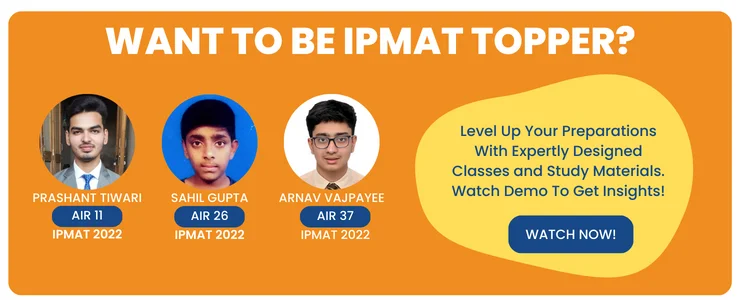Question 2: The number of acute-angled triangles whose sides are three consecutive positive integers and whose perimeter is at most 100 is

• 28
• 29
• 31
• 33

Solution.

We have been given three conditions:-

1. Triangle sides (a+b > c)
2. Acute angle triangle (a2 + b2 > c2)
3. Perimeter <= 100 (a + b + c <= 100)

Let's take random values for three consecutive integers: 1, 2, 3

This selection is impossible as it does not satisfy the first condition that the sum of two sides of a triangle should be greater than the third side. Therefore we rule this out. :  2, 3, 4

The first condition is satisfied, but the second condition, i.e., Acute angle property, is the sum of the square of any two sides has to be greater than the square of the third side 22 + 32 > 42

As 13 < 16. (So this condition is also not satisfied) : 3, 4, 5

The first condition is satisfied, but the second condition, i.e., Acute angle property, is the sum of the square of any two sides has to be greater than the square of the third side 32 + 42 > 52

As 25 = 25. (So this condition is also not satisfied) : 4, 5, 6

Since all the conditions mentioned above are satisfied, 4,5,6 will be our first consecutive integer set. Then (5,6,7) works and so on.

When the numbers become larger squares, they become increasingly larger

So after 4,5,6, all sets will satisfy condition 2.

Now we need to know the last possible consecutive number set based on the third condition.

Which gives 32,33,34 -Perimeter is at most 100

Therefore from the first 32 Combinations, the first 3 are ruled out. So in total, 29 combinations are possible.

Hence choice B is the correct answer.

Question3 : The value of cos2 /8+ cos2 3/8 + cos2 5/8 + cos2 7/8 is.

• 1
• 3/2
• 2
• 9/4

Solution.

We know that 58= 2 + 8

And 78= 2 + 38 =

=> cos2 /8+ cos2 3/8 + cos2 (/2 +/8 ) + cos2 (/2 +3/8)

=> cos2 /8+ cos2 3/8 + sin2 /8 + sin2 3/8

=> (cos2 /8+ sin2 /8 )+ (cos2 3/8 + sin2 3/8)

=> 1 + 1

=> 2

Hence the correct choice is option C.

Q4. if 112122+132+142+ ..... upto 26, then the value of 112+132+152+ ..... upto , is.

• 2/8
• 2/16
• 2/12
• 2/36

Solution.

Given 112+ 122+132+142+ ..... upto = 26 -(i)

Separation even and odd terms

(112+132+152+ ..... upto ) +(122+142+162+ ..... upto ) = 26

x + 1/4(112+ 122+132+142+ ..... upto ) = 26

x + 1/4(112+ 122+132+142+ ..... upto ) = 26

x + 1/4( 26) = 26 from (i)

x =26(1 - 14)

x =28

Hence the correct choice is option A.

Question 5: A 2 × 2 matrix is filled with four distinct integers randomly chosen from the set {1,2,3,4,5,6}, Then the probability that the matrix generated in such a way is singular is

• 2/45
• 1/45
• 4/15
• 1/15

Solution.

We know that the matrix will be singular only if its determinant is zero.

Therefore, the given matrix be

 a c b c

= |acdb| = ab - cd = 0, Or ab = cd

Using hit and trial from the given set {1,2,3,4,5,6}.

1. Let one of a,b,c or d is 5, then ab ≠ cd, because in the given set {1,2,3,4,5,6} there is only one multiple of 5. So now we have to select 4 integers from {1,2,3,4,6}
2. Let's take 3 or 6, since 6 is a multiple of 3 we will need to pick both of them. Therefore now the equation becomes 3b = 6d

Read more: Important Maths formulas for IPMAT exam

From the above equation we can infer that, b > d

{b, d} ⊂ {1, 2, 4}

If b = 4, d = 2

If b = 2, d = 1

Taking 3 and 6 we observed that only 2 choices were possible i.e {a, b, c, d} = {1, 2, 3, 6} or {a, b, c, d} = {4, 2, 3, 6}. Hence, we can select four numbers from the six in 6C4 = 15 ways

Therefore there will be 15 ways to select them and 4! ways to arrange them in the matrix.

So, the total number of matrices that can form is 15 x 4!.

Once we select {a, b, c, d} = {1, 2, 3, 6} or {a, b, c, d} = {4, 2, 3, 6}, for the matrix to be singular 2,3 or 4,3 should be on the same diagonal. Hence , only ⅓ of the arrangements yield a singular matrix.

Only one-third of the arrangements yield a singular matrix, and the others don’t.

Probability of getting a singular matrix = 2( 4! ⅓ )154! = 2/45.

Hence option A is the correct choice.

### Important Verbal Ability Questions for IPMAT 2023

Here is a sample question from the verbal ability section of the IPMAT Exam. Try solving this question to know how well you can attempt IPM Verbal Ability Questions.

Along with this question, you are advised to practice more questions from previous year's papers to test your preparation levels.

(Q.1 - 6)Read the following passage and choose the answer that is closest to each of the questions that are based on the passage.

Q1. When a dozen men are cast away on an imaginary island, the best educated will look for metals in rocks because

1. Metals can be used to make weapons.
2. Such an island probably has unexploited resources.
3. He may find it beneath him to dig or cut or make shoes.
4. He is suited for such work.

Q2. The author states that any appearance of secrecy or separateness would instantly and justly be looked upon with suspicion. From this statement, we may infer that

1. what is secret is not what is separate
2. secrecy is not the same as separateness
3. it is natural to be suspicious of the secrecy
4. it only takes an instant for a relationship to deteriorate

Q3. The instance of the shoemaker who refuses to show his source and asks for more corn and potatoes is an example of

1. a strong bargain.
2. Unfair practice.
3. The system of barter.
4. The intent to make trouble.

Q4. According to the author, whatever one's work might be

1. Hardships are going to be part of it.
2. One cannot keep complaining.
3. One should expect others to assure of help and advance our labours.
4. One must offer help to others in order to receive help.

Q5. The author's belief is that for progress to happen

1. A team should consist of people with multiple talents.
2. Cooperation among team members is essential.
3. One must deal with those who are secretive.
4. Transparency among all concerned is mandatory.

Q6. The writer makes a hypothesis, which can be related to

1. communities in general.
2. An imaginary island, rich with resources.
3. An ideal world of talented people.
4. A primitive and unsophisticated world.

Question: Rearrange the following sentences

• A. Despite the strong performance of the economy in 2010-11, the outlook for 2011-12 is clouded by stubborn and persistently high inflation and rising external risks.
• B. The three key macroeconomic concerns before the Union Budget 2011-12 were high inflation, high current account deficit (CAD), and fiscal consolidation.
• C. Additionally, there was an expectation that the government would restart the reform process.
• D. While the Budget sets a lower nominal gross domestic product (GDP) growth target of 14%, we believe that the real GDP growth target of 9% factored in the Budget is optimistic.
• E. The Budget has attempted to address all these issues, albeit through small steps.

2. CBAED

3. DACEB

With adequate preparation and strategy, you should be able to clear the IPMAT Exam on the first attempt. Joining a good coaching institute for guidance is recommended but not mandatory. In addition, referring to the appropriate books and study materials will aid in passing the exam with good marks.
To score good marks, candidates must follow expert preparation tips. Before you begin your preparation, review the complete test pattern and curriculum for the IPMAT Exam and create a comprehensive study plan. Study each topic by referring to the appropriate IPMAT Books, and make sure to finish studying the topics specified for each day without excuses. Participate in the Mock Test Series on a regular basis to assess your preparation and Solve previous year papers to determine the difficulty level of the paper and to estimate the different types of questions asked in the examination.
The question paper is divided into two categories, according to the IPMAT Syllabus: Quantitative Ability (Multiple Choice and Short Answer Questions) and Verbal Ability (Multiple Choice and Short Answer Questions) (Multiple Choice Questions). There are 40 questions in the Quantitative Ability Multiple Choice portion, and 20 questions in the Quantitative Ability Short Answer section. There are 40 multiple-choice questions in the Verbal Ability section.
• You can understand the exam's difficulty level.
• Recognize the successful attempts.
• Also, be aware of the types of questions that will be asked in each segment.
According to the IPMAT Exam Analysis, a good attempt depends upon various factors like difficulty level of exam, number of students appearing, etc. Generally speaking, anything between 65-70 correct attempts would be a good attempt.
• Home
• IPMAT Question Paper...

# IPMAT Question Paper Analysis & Solutions

Author : Palak Khanna

Updated On : January 27, 2023

SHARE

The Indian Institute of Management (IIM) conducts the Integrated Program in Management Aptitude Test (IPMAT) annually for providing admission to the five-year integrated program in management.

Going through the previous year's paper analysis will let you understand better the essential topics to focus on more for better results.

Our experts at Supergards have curated the IPMAT Exam Analysis and Solutions of the past five years in this post.

The takeaways from this post can immensely help you in streamlining your preparation to a result-oriented pathway and also get an idea about the number of questions asked from each subject, the difficulty level of the paper, type of questions, and more.

## IPMAT Exam Analysis Trend

The following points explain the Past Five-year's Exam Analysis of the IIM IPM Exam, such as mode of exam, sections involved, nature of questions, and more.

• Type of questions: Multiple Choice Questions MCQs and Short Answer (SA) questions.
• Sections: Generally, there are three sections in the exam. Two sections are of quantitative ability, and one is verbal ability.
• Maximum Marks: The is conducted for a total of 400 marks.
• Marking scheme: 4 marks are awarded for every correct answer, one mark is deducted for every wrong answer in MCQs, and there is no negative marking in short answers.

• Sectional Cut-off: It is applicable to all three sections.
• Difficulty Level: The overall difficulty level of IPMAT, considering previous years’ examination, is moderate to difficult.
• Verbal Ability: This is the most scoring section in the examination, with about 70-80% of questions from easy to moderate difficulty.
• Quantitative Ability: MCQ is generally the most challenging section, with about 40-50% moderate to complex questions.

### IPM Question Paper Analysis for Quantitative Ability (Short Answers)

As per the Exam Pattern for IPMAT, 50% of the question paper comprises the quantitative section (including MCQs and short answer-type questions).

• Generally, the SA section is of moderate difficulty having 20-30% tricky questions.
• Majorly, the questions are based on Logs, Arithmetic Progressions, Geometry, P&C, Probability.
• Statistically, 40% of questions are based on logarithms and Sequence & Series, and the same trend is followed from the past few years.
• A good attempt would be anything between 70-80% attempt with 80% accuracy.
• The pie chart plotted below represents an overall trend of the percentage of questions asked from different topics.Read more: Important topics for the IPMAT exam

### IPMAT Question Paper Analysis for Quantitative Ability (MCQ) Section

• Generally, this section is the most difficult section having 40-50% tricky questions and 40 questions to be completed within 40 minutes.
• Majorly questions are based on Number Systems, Arithmetic, Algebra, Geometry and Menstruation, P & C, Sets, Data Interpretation, and Matrices.
• Statistically, 50-55% of questions are based on Algebra, Arithmetics, and Data Interpretation.
• A good attempt would be anything between 65-70% attempt with 85% accuracy, fetching you about 80-90 marks.
• The below pie chart represents an overall trend of the percentage of questions asked from different topics.### IPM Question Paper Analysis for Verbal Ability Section

As per the previous year's trends, the IPMAT verbal ability section questions do not test your conventional English language or do not ask direct vocabulary questions like antonyms, synonyms, analogies, etc.

Instead, the verbal questions test your overall expertise in English with question types like usage of words & phrases, fill in the blanks, and your capability to use contextual vocabulary.

Check out the detailed IPMAT Question Paper analysis and solutions for the verbal ability section from the post below.

• Generally, this section is the easiest section having about 80% of questions of easy to moderate level difficulty.
• Majorly questions are based on Reading Comprehension, Fill in the blanks, Sentence Completion, Para Jumbles, and sentence completion-based questions.
• Statistically, 50-55% of questions are based on reading comprehension and fill-in blanks.
• A good attempt would be anything between 90% attempt with 85-90% accuracy, fetching you about 120-125 marks.
• The below pie chart represents an overall trend of the percentage of questions asked from different topics.Read more: IPMAT Fee structure and scholarships

### Important Questions with Solutions for IPMAT Quantitative Ability (Short Answer)

To help you get an idea about the type of questions asked in the quantitative aptitude (short answer) section, we have provided a few sample questions that were curated from the previous year's  IPMAT question papers.

Try to solve them for a better understanding of the difficulty level and the type of questions asked in the exam.

Question 1: In a division problem, the product of quotients and the remainder is 24 while their sum is 10. If the divisor is 5 then the dividend is __________. (IPMAT 2020)Solution 1 :

In this problem, we have given that the product of quotient(q) and the remainder(r) is 24.   i.e q x r = 24 -( i )

And it is also given that their sum is 10   i.e q + r = 10 -( ii )

Divisor(d) = 5.

( i ) and ( ii ) represent the sum and product of a quadratic equation, from ( ii ) putting r = q - 10, in ( i ), we will get q x (10 - q) = 24,    i.e  q2-10q + 24 = 0, which will give

q = 6 or 4.

Therefore one of them will be 6, and the other one will be 4. We do not know yet whether the quotient is 6 and the remainder is 4 or vice versa.  We know that  Dividend(n) = divisor(d) x quotient(q) + remainder(r) .

From the given data, n = 5 x q + r. - ( iii ),

As the divisor is 5, we infer that the remainder obviously will be smaller than 5. Therefore, the remainder will be 4, and the quotient will be 6. Putting the above-decided value in ( iii ).    n = 5(6) + 4 , therefore n = 34.

Question 2 : The shortest distance from the point (-4,3) to the circle x2 + y2 = 1 is __________. ( IPMAT 2020 )

Solution 2.

In this question, we have been given the equation of a circle and the coordinates of a point.

From the standard equation of circle i.e x2 + y2 = a2, we have a = 1.

The shortest distance of the point P(-4, 3) will be the distance from point P to the point where a straight line drawn from P to the circle's origin intersects the circle. As shown in the figure below, the shortest distance is PM.Question 3: The value ofQuestion 4: The minimum value of f(x)=|3-x|+|2+x|+|5-x| is equal to __________.

Solution.

Form f(x)=|3-x|+|2+x|+|5-x| We know that critical points would be x = 3 , x = -2 , and x = 5.

The slope of the line changes at these critical points.

ExampleNow we need to select the critical point which has the lowest value of y axis, from the figure above f(3) will have the lowest value.

f(3) = |3 - 3|+|2 + 3|+| 5 - 3|

f(3) = 0 + 5 + 2 .

f(3) = 7.

Question 5: Ashok purchased pens and pencils in the ratio 2: 3 during his first visit and paid Rs. 86 to the shopkeeper. During his second visit, he purchased pens and pencils in the ratio of 4: 1 and paid Rs. 112. The cost of a pen, as well as a pencil in rupees, is a positive integer. If Ashok purchased four pens during his second visit, then the amount he paid in rupees for the pens during the second visit is __________. (IPMAT 2020)

Solution.

We have been given that when the person spends Rs. 86, the ratio of pen and pencil is 2:3.

And when he pays Rs. 112, the ratio of pen and pencil is 4:1. And in this visit, the total number of pens is 4.

Let x be the price per pen and y be the price per pencil.

Therefore we have

4x + y = 112

2x + 3y = 86 (From first visit)

It is in Ratios, so it can be 4x + 6y = 86 (or)

6x + 9y = 86 (or)

8x + 12y = 86 and so on.

As we know, 4x + y = 112, we can infer that 4x + 6y = 86 is not possible, and hence all the equations after this are not possible.

So now we have 4x + y = 112

2x + 3y = 86

Solving the above equation we will get y = 12.

Therefore 4x + 12 = 112

=> 4x = cost of pens = 112 -12 = 100

Question 6: In a four-digit number, the product of thousands digit and units digit is zero while their difference is 7. The product of the middle digits is 18. The thousands digit is as much more than the units digit as the hundreds digit is more than the tens digit. The four-digit number is __________.

Solution.

Let the four-digit number be ABCD. D is the unit place number, C is the tens digit number, B is the hundreds digit number, and A is the thousand digit number.

Given data

1.  A x D = 0

This implies one of them will be zero; as A is the rightmost place, its value cannot be zero.

Therefore D = 0.

1. A - D = 7

As D = 0, A will be 7.

1. B x C = 18

B and C can hold values from 0-9 only; looking at the possible combinations, BC can be 92( or 29) or 63(or 36).

1. Given that the AD difference is the same as the difference of BC, therefore, B is 9 and C is 2.

Question 7: Two friends run a 3-kilometer race along a circular course of length 300 meters. If their speeds are in the ratio 3:2, the number of times the winner passes the other is __________.

Solution.

Let the speed of the fast one be 3x, and the speed of the slow one be 2x.

Time required for the fast one to cover one complete round = tf = 300/3x

Time required for the slow one to cover one complete round = ts = 300/2x

For finding out the time at which both meet for the first time, we need to find lcm of tf and ts

Tmeet = 300/x (lcm of tf and ts)

Distance traveled by the fast one when they meet = Tmeet * speed of fast one

= 300/x * 3x

= 900 m

Therefore they will first meet when the fast one has covered 900m; then they will meet at 1800, and then for the last at 2700 meters.

Therefore the winner will pass the slow one precisely 3 times.

### Important questions for IPMAT Quantitative Ability (MCQ)

In this section, the difficulty level of questions will be easy to moderate level of difficulty. Therefore, learning Short tricks to Attempt Quantitative Aptitude Section will help you fetch more marks than others.

Check out the questions that can be asked from the quantitative ability MCQ section from the post below.

Question 1: The probability that a randomly chosen factor of 1019 is a multiple of 1015 is

• 1/25
• 1/12
• 1/20
• 1/16

Solution.

Given number is 1019 , the factor of the given number are 219 x 519

Therefore the total factors of 1019 are (19 + 1)(19 + 1) = 400.

Multiples of 1015 are 215 x 515; This is of the form 2x × 5y

We need multiple numbers of 2x × 5y and a factor of 219 × 519. Therefore the possible values of x = 15 , 16 , 17 ,18 , 19

And possible values of y = 15 , 16 , 17 ,18 , 19

Therefore there are five possible values of x and five possible values of y.

Favourable outcome = 5 * 5 = 25

Total number of outcomes = 400

Probability = 25/400 = 1/16.

Hence the correct option is option D.Question 2: The number of acute-angled triangles whose sides are three consecutive positive integers and whose perimeter is at most 100 is

• 28
• 29
• 31
• 33

Solution.

We have been given three conditions:-

1. Triangle sides (a+b > c)
2. Acute angle triangle (a2 + b2 > c2)
3. Perimeter <= 100 (a + b + c <= 100)

Let's take random values for three consecutive integers: 1, 2, 3

This selection is impossible as it does not satisfy the first condition that the sum of two sides of a triangle should be greater than the third side. Therefore we rule this out. :  2, 3, 4

The first condition is satisfied, but the second condition, i.e., Acute angle property, is the sum of the square of any two sides has to be greater than the square of the third side 22 + 32 > 42

As 13 < 16. (So this condition is also not satisfied) : 3, 4, 5

The first condition is satisfied, but the second condition, i.e., Acute angle property, is the sum of the square of any two sides has to be greater than the square of the third side 32 + 42 > 52

As 25 = 25. (So this condition is also not satisfied) : 4, 5, 6

Since all the conditions mentioned above are satisfied, 4,5,6 will be our first consecutive integer set. Then (5,6,7) works and so on.

When the numbers become larger squares, they become increasingly larger

So after 4,5,6, all sets will satisfy condition 2.

Now we need to know the last possible consecutive number set based on the third condition.

Which gives 32,33,34 -Perimeter is at most 100

Therefore from the first 32 Combinations, the first 3 are ruled out. So in total, 29 combinations are possible.

Hence choice B is the correct answer.

Question3 : The value of cos2 /8+ cos2 3/8 + cos2 5/8 + cos2 7/8 is.

• 1
• 3/2
• 2
• 9/4

Solution.

We know that 58= 2 + 8

And 78= 2 + 38 =

=> cos2 /8+ cos2 3/8 + cos2 (/2 +/8 ) + cos2 (/2 +3/8)

=> cos2 /8+ cos2 3/8 + sin2 /8 + sin2 3/8

=> (cos2 /8+ sin2 /8 )+ (cos2 3/8 + sin2 3/8)

=> 1 + 1

=> 2

Hence the correct choice is option C.

Q4. if 112122+132+142+ ..... upto 26, then the value of 112+132+152+ ..... upto , is.

• 2/8
• 2/16
• 2/12
• 2/36

Solution.

Given 112+ 122+132+142+ ..... upto = 26 -(i)

Separation even and odd terms

(112+132+152+ ..... upto ) +(122+142+162+ ..... upto ) = 26

x + 1/4(112+ 122+132+142+ ..... upto ) = 26

x + 1/4(112+ 122+132+142+ ..... upto ) = 26

x + 1/4( 26) = 26 from (i)

x =26(1 - 14)

x =28

Hence the correct choice is option A.

Question 5: A 2 × 2 matrix is filled with four distinct integers randomly chosen from the set {1,2,3,4,5,6}, Then the probability that the matrix generated in such a way is singular is

• 2/45
• 1/45
• 4/15
• 1/15

Solution.

We know that the matrix will be singular only if its determinant is zero.

Therefore, the given matrix be

 a c b c

= |acdb| = ab - cd = 0, Or ab = cd

Using hit and trial from the given set {1,2,3,4,5,6}.

1. Let one of a,b,c or d is 5, then ab ≠ cd, because in the given set {1,2,3,4,5,6} there is only one multiple of 5. So now we have to select 4 integers from {1,2,3,4,6}
2. Let's take 3 or 6, since 6 is a multiple of 3 we will need to pick both of them. Therefore now the equation becomes 3b = 6d

Read more: Important Maths formulas for IPMAT exam

From the above equation we can infer that, b > d

{b, d} ⊂ {1, 2, 4}

If b = 4, d = 2

If b = 2, d = 1

Taking 3 and 6 we observed that only 2 choices were possible i.e {a, b, c, d} = {1, 2, 3, 6} or {a, b, c, d} = {4, 2, 3, 6}. Hence, we can select four numbers from the six in 6C4 = 15 ways

Therefore there will be 15 ways to select them and 4! ways to arrange them in the matrix.

So, the total number of matrices that can form is 15 x 4!.

Once we select {a, b, c, d} = {1, 2, 3, 6} or {a, b, c, d} = {4, 2, 3, 6}, for the matrix to be singular 2,3 or 4,3 should be on the same diagonal. Hence , only ⅓ of the arrangements yield a singular matrix.

Only one-third of the arrangements yield a singular matrix, and the others don’t.

Probability of getting a singular matrix = 2( 4! ⅓ )154! = 2/45.

Hence option A is the correct choice.

### Important Verbal Ability Questions for IPMAT 2023

Here is a sample question from the verbal ability section of the IPMAT Exam. Try solving this question to know how well you can attempt IPM Verbal Ability Questions.

Along with this question, you are advised to practice more questions from previous year's papers to test your preparation levels.

(Q.1 - 6)Read the following passage and choose the answer that is closest to each of the questions that are based on the passage.

Q1. When a dozen men are cast away on an imaginary island, the best educated will look for metals in rocks because

1. Metals can be used to make weapons.
2. Such an island probably has unexploited resources.
3. He may find it beneath him to dig or cut or make shoes.
4. He is suited for such work.

Q2. The author states that any appearance of secrecy or separateness would instantly and justly be looked upon with suspicion. From this statement, we may infer that

1. what is secret is not what is separate
2. secrecy is not the same as separateness
3. it is natural to be suspicious of the secrecy
4. it only takes an instant for a relationship to deteriorate

Q3. The instance of the shoemaker who refuses to show his source and asks for more corn and potatoes is an example of

1. a strong bargain.
2. Unfair practice.
3. The system of barter.
4. The intent to make trouble.

Q4. According to the author, whatever one's work might be

1. Hardships are going to be part of it.
2. One cannot keep complaining.
3. One should expect others to assure of help and advance our labours.
4. One must offer help to others in order to receive help.

Q5. The author's belief is that for progress to happen

1. A team should consist of people with multiple talents.
2. Cooperation among team members is essential.
3. One must deal with those who are secretive.
4. Transparency among all concerned is mandatory.

Q6. The writer makes a hypothesis, which can be related to

1. communities in general.
2. An imaginary island, rich with resources.
3. An ideal world of talented people.
4. A primitive and unsophisticated world.

Question: Rearrange the following sentences

• A. Despite the strong performance of the economy in 2010-11, the outlook for 2011-12 is clouded by stubborn and persistently high inflation and rising external risks.
• B. The three key macroeconomic concerns before the Union Budget 2011-12 were high inflation, high current account deficit (CAD), and fiscal consolidation.
• C. Additionally, there was an expectation that the government would restart the reform process.
• D. While the Budget sets a lower nominal gross domestic product (GDP) growth target of 14%, we believe that the real GDP growth target of 9% factored in the Budget is optimistic.
• E. The Budget has attempted to address all these issues, albeit through small steps.

2. CBAED

3. DACEB

With adequate preparation and strategy, you should be able to clear the IPMAT Exam on the first attempt. Joining a good coaching institute for guidance is recommended but not mandatory. In addition, referring to the appropriate books and study materials will aid in passing the exam with good marks.
To score good marks, candidates must follow expert preparation tips. Before you begin your preparation, review the complete test pattern and curriculum for the IPMAT Exam and create a comprehensive study plan. Study each topic by referring to the appropriate IPMAT Books, and make sure to finish studying the topics specified for each day without excuses. Participate in the Mock Test Series on a regular basis to assess your preparation and Solve previous year papers to determine the difficulty level of the paper and to estimate the different types of questions asked in the examination.
The question paper is divided into two categories, according to the IPMAT Syllabus: Quantitative Ability (Multiple Choice and Short Answer Questions) and Verbal Ability (Multiple Choice and Short Answer Questions) (Multiple Choice Questions). There are 40 questions in the Quantitative Ability Multiple Choice portion, and 20 questions in the Quantitative Ability Short Answer section. There are 40 multiple-choice questions in the Verbal Ability section.
• You can understand the exam's difficulty level.
• Recognize the successful attempts.
• Also, be aware of the types of questions that will be asked in each segment.
According to the IPMAT Exam Analysis, a good attempt depends upon various factors like difficulty level of exam, number of students appearing, etc. Generally speaking, anything between 65-70 correct attempts would be a good attempt.